# Mathematical Web Resources

 Page Index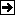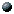About This PageMathematical Associations and SocietiesMathematical HistoryMathematical JournalsMathematical Services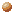Global Mathematical ServicesMathematical Services on Special SubjectsMathematical DatabasesMathematical Software

This page contains links to all kinds of mathematical resources on the web: societies and associations, journals, services, literature databases, problem and puzzle corners, fun stuff and jokes, games, software, art, history and much more.
Whereas mathematical professionals or deeply interested amateurs may be interested in associations, journals, mathematicel web services, and literature databases, others may find some helpful stuff concerning mathematical puzzles, jokes, games and art.

 Mathematical Associations and Societies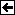Mathematical History•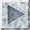History of Mathematics (part of www.mathpages.com)
is a collection of concise essays on several subjects concerning the history of mathematics.
•Mathematics - Ancient Science and Its Modern Fates
have a walk through the mathematics history part of the Library of Congress Vatican Exhibit
•HISTORIA MATEMATICA Forum (by Julio Gonzalez Cabillon)
is a closed mailing list "to provide a virtual forum for scholarly discussion of the HISTORY OF MATHEMATICS (in a broad sense), amongst professionals, and non-professionals with a serious interest in the field".

 Mathematical JournalsThis list reflects my former research interests (discrete mathematics, esp. graph theory), hence most of the journals deal with combinatorics and graph theory.

•Abhandlungen aus dem Mathematischen Seminar der Universität Hamburg (German/ published byMathematisches Seminar of Univ. Hamburg)
•aequationes mathematicae (AEM)
- "an international journal of pure and applied mathematics, which emphasizes functional equations, dynamical systems and combinatorics"
- printed and electronic edition available
- publisher:Birkhäuser)
•algebra universalis (AU) (covers "universal algebra, lattice theory and related fields"/ printed and electronic edition/ published byBirkhäuser)
•Annals of Combinatorics (publishes "outstanding contributions to combinatorial mathematics in all its respects"/ published byBirkhäuser)
•Ars Combinatoria (A Canadian Journal of Combinatorics)
•Combinatorics, Probability & Computing
•Discrete Applied Mathematics (The Journal of Combinatorial Algorithms, Informatics and Computer Sciences/ published byElsevier)
•Discrete Mathematics (brings together "research papers in different parts of discrete mathematics. The journal presents papers in combinatorial mathematics and related areas. The fields covered by Discrete Mathematics include in particular: graph and hypergraph theory, network theory, coding theory, block designs, lattice theory, the theory of partially ordered sets, combinatorial geometrics, matroid theory, extremal set theory, logic and automata, matrices, polyhedra, and discrete probability theory."/ published byElsevier)
•Discrete Mathematics & Theoretical Computer Science (DMTCS) an electronic journal
•Discussiones Mathematicae. Differential Inclusions, Control and Optimization (published by University Press Zielona Góra, Poland)
•Discussiones Mathematicae - General Algebra and Applications (published by University Press Zielona Góra, Poland)
•Discussiones Mathematicae Graph Theory (published by University Press Zielona Góra, Poland)
•Discussiones Mathematicae Probability and Statistics (published by University Press Zielona Góra, Poland)
•European Journal of Combinatorics ("international, bimonthly journal of pure mathematics, specializing in theories arising from combinatorial problems. The journal is primarily open to papers dealing with mathematical structures within combinatorics and/or establishing direct links between combinatorics and other branches of mathematics and the theories of computing."/ published byElsevier)
•Graphs and Combinatorics ("international journal devoted to research concerning all aspects of combinatorial mathematics"/ published bySpringer)
•Journal of Algebraic Combinatorics ("provides a single forum for papers on algebraic combinatorics which, at present, are distributed throughout a number of journals. Within the last decade or so, algebraic combinatorics has evolved into a mature, established and identifiable area of mathematics. Research contributions in the field are increasingly seen to have substantial links with other areas of mathematics."/ published bySpringer)
•Journal of Combinatorial Designs ("international journal devoted to the timely publication of the most influential papers in the area of combinatorial design theory. All topics in design theory, and in which design theory has important applications, are covered, including block designs, t-designs, pairwise balanced designs and group divisible designs; Latin squares, quasigroups, and related algebras; computational methods in design theory; construction methods; applications in computer science, experimental design theory, and coding theory; graph decompositions, factorizations, and design-theoretic techniques in graph theory and extremal combinatorics; finite geometry and its relation with design theory; algebraic aspects of design theory"/ published byWiley InterScience)
•Journal of Combinatorial Theory, Series B ("publishes original mathematical research dealing with theoretical and physical aspects of the study of finite and discrete structures in all branches of science. Series B is concerned primarily with graph theory and matroid theory (...)."/ published byElsevier)
•Journal of Discrete Mathematical Sciences & Cryptography (published byTaru Publications)
•Journal of Graph Algorithms and Applications (includes electronic version)
•Journal of Graph Theory ("devoted to a variety of topics in graph theory, such as structural results about graphs, graph algorithms with theoretical emphasis, and discrete optimization on graphs. The scope of the journal also includes related areas in combinatorics and the interaction of graph theory with other mathematical sciences."/ published byWiley InterScience)
•Journal of Information & Optimization Sciences (published byTaru Publications)
•Journal of Interdisciplinary Mathematics (published byTaru Publications)
•Journal of Statistics & Management Systems (published byTaru Publications)
•SIAM Journal on Discrete Mathematics ("publishes research articles on a broad range of topics from pure and applied mathematics including combinatorics and graph theory, discrete optimization and operations research, theoretical computer science, and coding and communication theory."/ published bySIAM Journals)
•The Electronic Journal of Combinatorics ("a fully-refereed electronic journal that welcomes papers in all branches of discrete mathematics, including combinatorics, graph theory, discrete algorithms, etc.")
•Zentralblatt MATH ("the world's most complete and longest running abstracting and reviewing service in pure and applied mathematics. TheZentralblatt MATH Database contains more than 2.0 million entries drawn from more than 2300 serials and journals and covers the period from 1868 - present by the recent integration of the Jahrbuch database (JFM).")

 Mathematical ServicesGlobal Mathematical Services•Portal: Mathematik (German/ byWikipedia)
•The European Mathematical Information Service (EMIS) (by the European Mathematical Society)
•Math-Net Links to the Mathematical World (by Konrad-Zuse-Zentrum für Informationstechnik Berlin (ZIB))
•MathPages cover the following fields: Number Theory, Combinatorics, Geometry, Algebra, Calculus and Differential Equations, Probability and Statistics, Set Theory and Foundations, Reflections on Relativity, History, Physics, Music, Animated Illustrations, Quotations. The site is huge, with hundreds of lengthy articles and essays. Strangely, no author or organization takes open responsibility for the pages. I read some of the articles - as far as I can see, they have a good quality.
•MathWorld (by Eric W. Weisstein)
"is a comprehensive and interactive mathematics encyclopedia intended for students, educators, math enthusiasts, and researchers."
•A Catalog of Mathematics Resources on the WWW and the Internet (by M. Maheswaran)
a huge collection of useful links
 Mathematical Services on Special SubjectsMathematical Databases•Zentralblatt MATH Database (by the European Mathematical Society)
retriever for the 'Zentralblatt für Mathematik/ Mathematics Abstracts'

 Mathematical Software•Andrew Goldberg's Network Optimization Library
several software packages to run under UNIX
•ChaosPro
"is a freeware fractal generator for the Microsoft Windows operating system, able to create beautiful images based on mathematical formulas." ChaosPro is an extensive program, with zoom function and many other features.download page
•Euler
"is a powerful numerical laboratory with a programming language. The system can handle real, complex and interval numbers, vectors and matrices. It can produce 2D/3D plots. (...) Euler comes with Yacas, a computer algebra system. (...) All versions are freeware and open source under the GNU general license."download page
•nauty (by Brendan McKay)
is a package to compute the automorphism group of graphs and digraphs.
•YACAS (yet another computer algebra system)
"is an easy to use, general purpose Computer Algebra System, a program for symbolic manipulation of mathematical expressions. It uses its own programming language designed for symbolic as well as arbitrary-precision numerical computations." YACAS is open source.download page